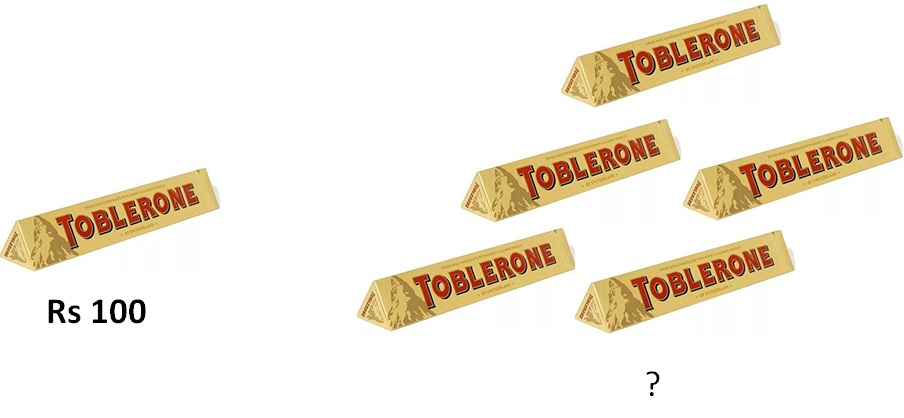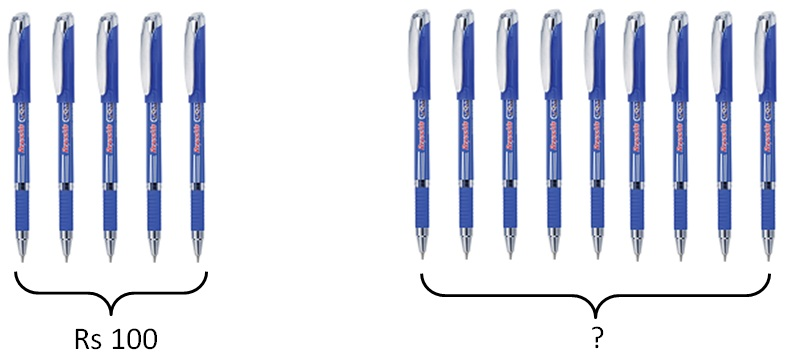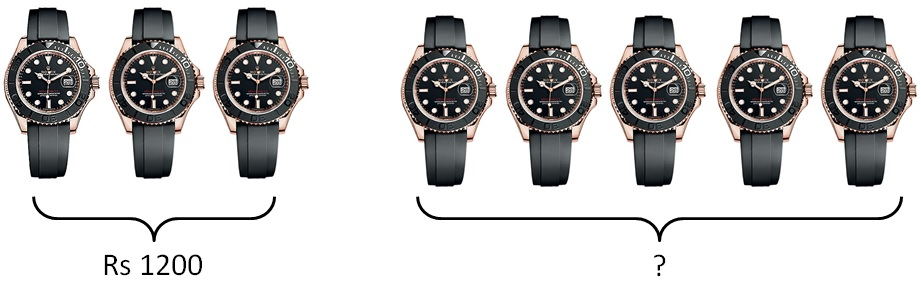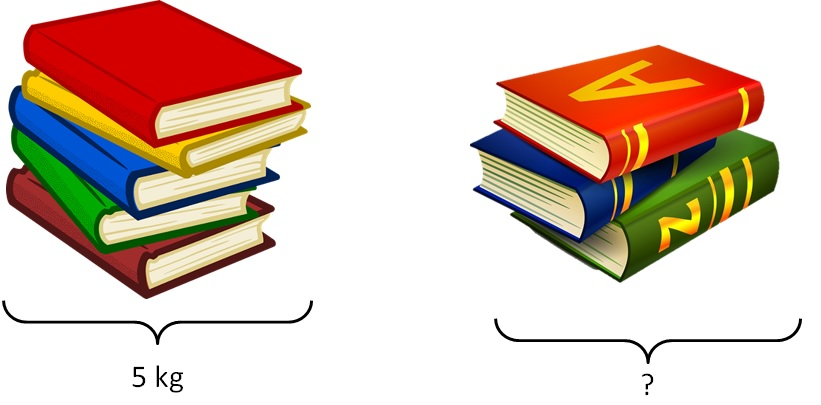Unitary Method

Chapter 12 Class 6 Ratio and Proportion
Concept wise

Suppose 1 chocolate costs Rs 100

How much would 5 chocolate cost?Cost of 1 Chocolate = Rs 100

Cost of 5 Chocolate = Rs 5 × 100

= Rs 500

Let’s take another example

#### Suppose 5 pens cost Rs 100. How would 9 pen cost?Cost of 5 pens = Rs 100

Cost of 1 pen = Rs 100/5

Cost of 9 pens = Rs 9 × 100/5

= 9 × 20

= Rs 180

So,

What we did was,

We find cost of 1 pen, & then find cost of 9 pens

This method of finding value of 1 unit

& then using it to find value of multiple units is called Unitary Method.

Let’s take another example

#### Three watches cost Rs 1200. How much five watches would cost?Cost of three watches = Rs 1200

Cost of one watch = Rs 1200/3

Cost of five watches = Rs 1200/3 × 5

= 400 × 5

= Rs 2000

#### 5 identical books weights 5 kg. How much 3 of these books weight?Weight of 5 identical books = Rs 5 kg

Weight of 1 books = 5/5 kg

Weight of 1 books = 5/5 kg × 3 kg

= 1 × 3

= 3 kg

#### A train takes 3 hours to travel 180 km. How much time would it take to travel 540 km?

Time taken to travel 180 km = 3 hours

Time taken to travel 1 km = 3/180 hours

Time taken to travel 540 km = 3/180 × 540 hours

= 3/18 × 54 hours

= 1/6 × 54 hours

= 9 hours

Learn in your speed, with individual attention - Teachoo Maths 1-on-1 Class